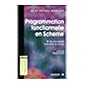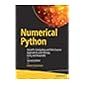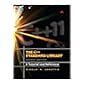Normal view

# Numerical Python : scientific computing and data science applications with Numpy, SciPy and Matplotlib / Robert, Johansson [ Livre]

Langue: Anglais ; de l'oeuvre originale, Anglais.Mention d'édition: Second editionPublication : New York : Apress, 2019Description : 1 vol. (XXIII-700 p.) ; 26 cmISBN: 9781484242452.Classification: 005.13 Langages de programmationRésumé: La 4ème de couv. indique : "Leverage the numerical and mathematical modules in Python and its standard library as well as popular open source numerical Python packages like NumPy, SciPy, FiPy, matplotlib and more. This fully revised edition, updated with the latest details of each package and changes to Jupyter projects, demonstrates how to numerically compute solutions and mathematically model applications in big data, cloud computing, financial engineering, business management and more. Numerical Python, Second Edition, presents many brand-new case study examples of applications in data science and statistics using Python, along with extensions to many previous examples. Each of these demonstrates the power of Python for rapid development and exploratory computing due to its simple and high-level syntax and multiple options for data analysis. After reading this book, readers will be familiar with many computing techniques including array-based and symbolic computing, visualization and numerical file I/O, equation solving, optimization, interpolation and integration, and domain-specific computational problems, such as differential equation solving, data analysis, statistical modeling and machine learning.".Sujet - Nom commun:
Current location Call number Status Notes Date due Barcode
ENS Rennes - Bibliothèque
Informatique
005.13 JOH (Browse shelf) Available 005.13 Langages de programmation 043398
##### Browsing ENS Rennes - Bibliothèque shelves, Shelving location: Informatique Close shelf browserNo cover image available005.13 HAV Eloquent JavaScript : a Modern Introduction to Programming 005.13 HER Apprenez à programmer en Java 005.13 HUF Programmation fonctionnelle en Scheme : de la conception à la mise en oeuvre 005.13 JOH Numerical Python : scientific computing and data science applications with Numpy, SciPy and Matplotlib 005.13 JOS The C++ standard library : a tutorial and reference 005.13 JOS The C++ standard library : a tutorial and reference 005.13 KER Le langage C : norme ANSI

La 4ème de couv. indique : "Leverage the numerical and mathematical modules in Python and its standard library as well as popular open source numerical Python packages like NumPy, SciPy, FiPy, matplotlib and more. This fully revised edition, updated with the latest details of each package and changes to Jupyter projects, demonstrates how to numerically compute solutions and mathematically model applications in big data, cloud computing, financial engineering, business management and more. Numerical Python, Second Edition, presents many brand-new case study examples of applications in data science and statistics using Python, along with extensions to many previous examples. Each of these demonstrates the power of Python for rapid development and exploratory computing due to its simple and high-level syntax and multiple options for data analysis. After reading this book, readers will be familiar with many computing techniques including array-based and symbolic computing, visualization and numerical file I/O, equation solving, optimization, interpolation and integration, and domain-specific computational problems, such as differential equation solving, data analysis, statistical modeling and machine learning."